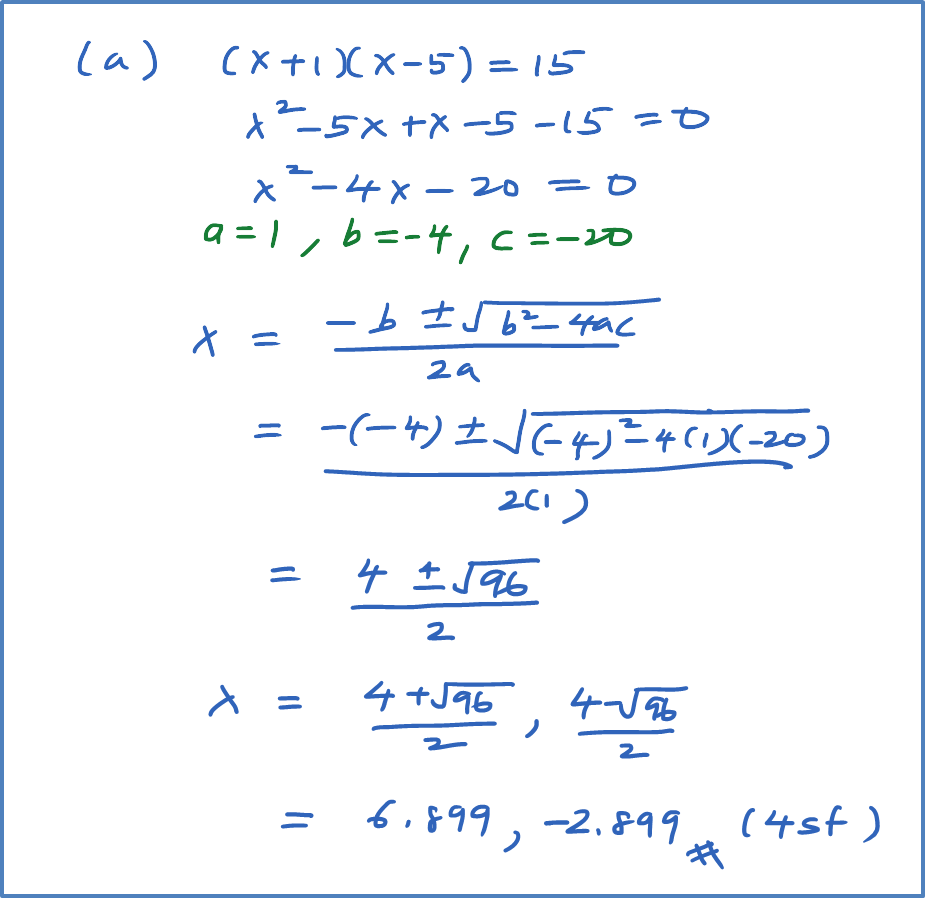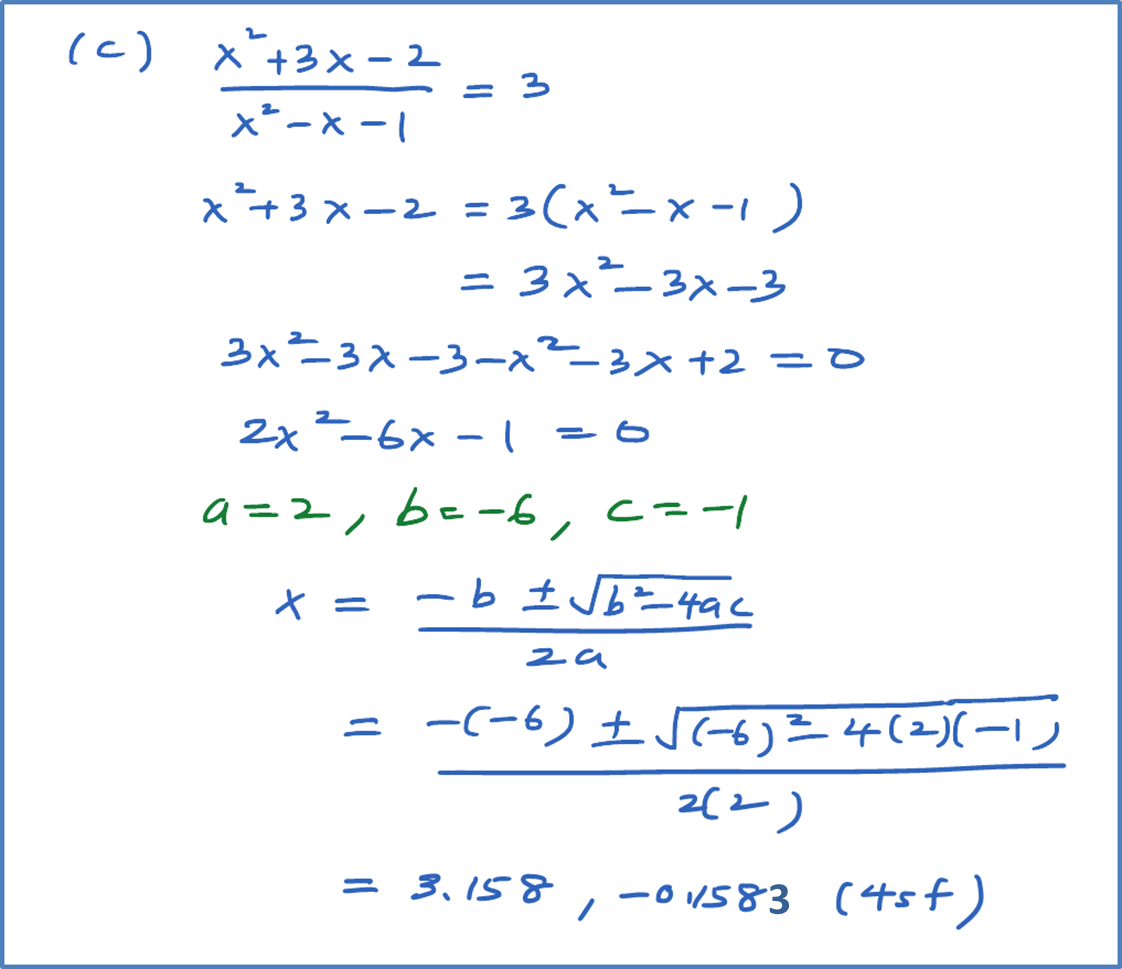\

# 2.12.1 Quadratic Functions, SPM Practice (Paper 1)

Question 1:
Solve the following quadratic equations by factorisation.

Solution:Question 2:
Solve the following quadratic equations by completing the square.

Solution:Question 3: